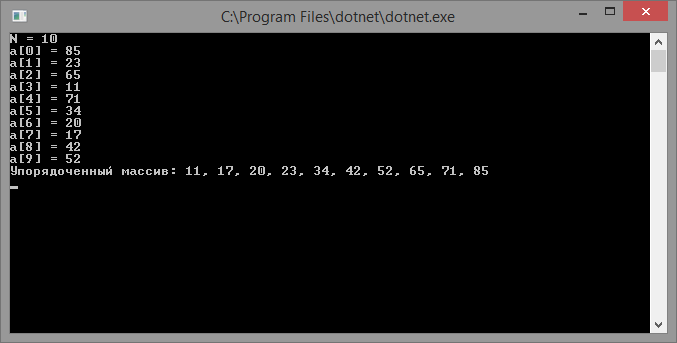# Quick sort

Updated: 15.09.2019

Quick sort or Hoar sort is one of the fastest data sorting algorithms.

The Hoar Algorithm is a modified version of the direct exchange method. Other popular variations of this method - bubble sort and shaker sort , unlike quick sort, are not very effective.

## The principle of operation of the quick sort algorithm

The idea of ​​the algorithm is as follows:

• It is necessary to choose a supporting element of the array, it can be any element, the correctness of the algorithm does not depend on this;
• Divide the array into three parts, the first should include elements less than reference, the second - equal to the reference and the third - all elements are larger than the reference;
• The previous steps are performed recursively, for subarrays with smaller and larger values, as long as they contain more than one element.

Since the quick sort method divides the array into parts, it belongs to the group of algorithms divide and conquer.

## Implement quick sort

``````using System;

class program
{
// method for exchanging array elements
static void Swap(ref int x, ref int y)
{
var t = x;
x = y;
y = t;
}

// method returns the index of the reference element
static int Partition(int[] array, int minIndex, int maxIndex)
{
var pivot = minIndex - 1;
for (var i = minIndex; i < maxIndex; i++)
{
if (array[i] < array[maxIndex])
{
pivot++;
Swap(ref array[pivot], ref array[i]);
}
}

pivot++;
Swap(ref array[pivot], ref array[maxIndex]);
return pivot;
}

// quick sort
static int[] QuickSort(int[] array, int minIndex, int maxIndex)
{
if (minIndex >= maxIndex)
{
return array;
}

var pivotIndex = Partition(array, minIndex, maxIndex);
QuickSort(array, minIndex, pivotIndex - 1);
QuickSort(array, pivotIndex + 1, maxIndex);

return array;
}

static int[] QuickSort(int[] array)
{
return QuickSort(array, 0, array.Length - 1);
}

static void Main(string[] args)
{
Console.Write("N =");
var a = new int[len];
for (var i = 0; i < a.Length; ++i)
{
Console.Write("a [{0}] =", i);
}

Console.WriteLine("Ordered array: {0}", string.Join(",", QuickSort(a)));

The result of the program: